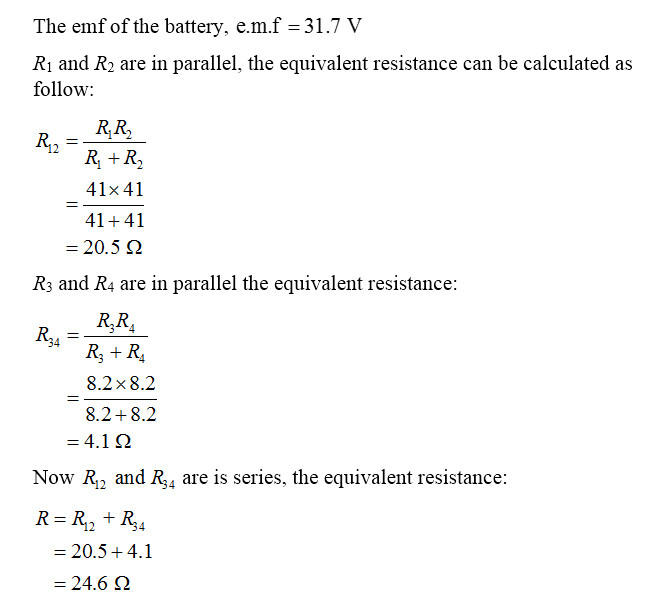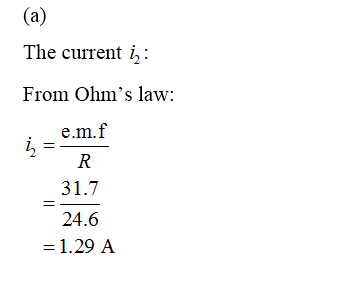Question
12 views

In the figure the ideal battery has emf = 31.7 V, and the resistances are R1 = R2 = 41 Ω, R3 = R4 = R5 = 8.2 Ω, R6 = 5.4 Ω, and R7 = 4.8 Ω. What are currents (a)i2, (b)i4, (c)i1, (d)i3, and (e)i5?

check_circle

Step 1

We’ll answer the first three subparts of the question since the exact one wasn’t specified. Please submit a new question specifying the one you’d like answered

Step 2Step 3...

### Want to see the full answer?

See Solution

#### Want to see this answer and more?

Solutions are written by subject experts who are available 24/7. Questions are typically answered within 1 hour.*

See Solution
*Response times may vary by subject and question.
Tagged in